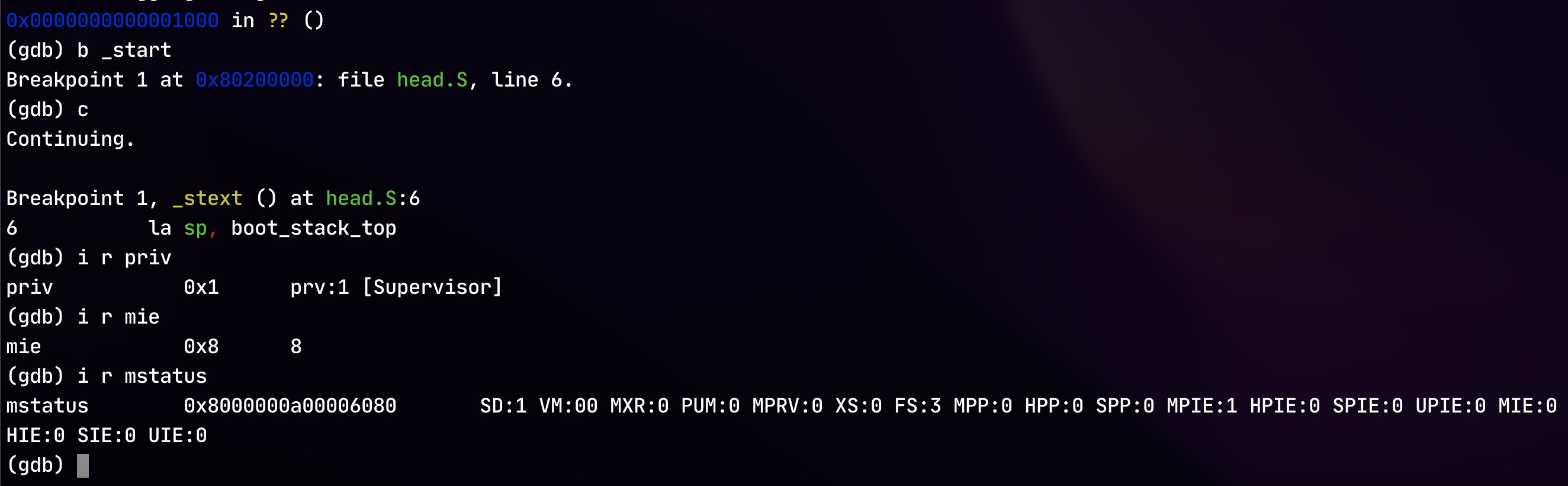# RV64 内核引导 ¶

1193 个字 112 行代码 预计阅读时间 5 分钟

Abstract

Warning

## 实验内容 ¶

• 编写、完成所给实验代码框架，并成功运行
• lib/Makefile
• arch/riscv/kernel/sbi.c
• lib/print.c
• arch/riscv/include/defs.h
• 思考题
1. 编译之后，通过 System.map 查看 vmlinux.lds 中自定义符号的值，比较他们的地址是否符合你的预期
2. 在你的第一条指令处添加断点，观察你的程序开始执行时的特权态是多少，中断的开启情况是怎么样的
3. 在你的第一条指令处添加断点，观察内存中 text、data、bss 段的内容是怎样的
4. 尝试从汇编代码中给 C 函数 start_kernel 传递参数

## 代码编写 ¶

head.S 的作用是作为整个内核启动的引导。其要完成的是 1. start_kernel 设置一个栈空间（即创建栈空间并将 sp 指到栈顶）2. 跳转到 start_kernel 内。

vmlinux.lds 的作用是定义内核的内存布局。我们的目的是将栈空间放到 _end 后面，所以可以在 vmlinux.lds _end 后面再加一个段 .stack，方便后续 head.S 在此处插入栈空间：

    _end = .;

.stack : ALIGN(0x1000){
_sstack = .;

*(.stack.entry)

_estack = .;
}
}


.extern start_kernel

.section .text.entry
.globl _start
_start:
la sp, boot_stack_top
j start_kernel

.section .stack.entry
.globl boot_stack_bottom
boot_stack_bottom:
.space 4096
.globl boot_stack_top
boot_stack_top:


### sbi.c¶

sbi.c 的作用是实现 SBI 调用。最主要的目的是实现 sbi_ecall 来执行环境调用，用其可以实现 sbi_console_putchar 等函数。

sbi_ecall 的实现参考了 Linux 6.0.7 的代码实现。其主要过程就是将函数参数布局到寄存器中（ext 放到 a7fid 放到 a6arg0-5 放到 a0-5），然后执行 ecall 指令，最后返回的结果有 a0 表示 error codea1 表示返回值（所以这两个寄存器要是读写的，其它是可读即可），并且可能会修改内存。利用内联汇编可以进行如下实现：

struct sbiret sbi_ecall(int ext, int fid, uint64 arg0,
uint64 arg1, uint64 arg2,
uint64 arg3, uint64 arg4,
uint64 arg5)
{
struct sbiret ret;
register uint64 a0 asm("a0") = (uint64)(arg0);
register uint64 a1 asm("a1") = (uint64)(arg1);
register uint64 a2 asm("a2") = (uint64)(arg2);
register uint64 a3 asm("a3") = (uint64)(arg3);
register uint64 a4 asm("a4") = (uint64)(arg4);
register uint64 a5 asm("a5") = (uint64)(arg5);
register uint64 a6 asm("a6") = (uint64)(fid);
register uint64 a7 asm("a7") = (uint64)(ext);
asm volatile (
"ecall"
: "+r" (a0), "+r" (a1)
: "r" (a2), "r" (a3), "r" (a4), "r" (a5), "r" (a6), "r" (a7)
: "memory"
);
ret.error = a0;
ret.value = a1;
return ret;
}


void sbi_set_timer(uint64 stime_value) {
sbi_ecall(0x00, 0, stime_value, 0, 0, 0, 0, 0);
}

void sbi_console_putchar(int ch) {
sbi_ecall(0x01, 0, ch, 0, 0, 0, 0, 0);
}

int sbi_console_getchar() {
struct sbiret ret;
ret = sbi_ecall(0x02, 0, 0, 0, 0, 0, 0, 0);
return ret.error;
}


### print.c & Makefile¶

print.c 中需要定义两个使用 sbi_ecall (sbi_console_putchar) 来实现输出的两个函数 puts puti。其中 puts 直接逐字符调用 sbi_console_putchar 输出直到遇到 '\0'

void puts(char *s) {
while (*s) {
sbi_console_putchar(*s++);
}
}

puti 先枚举 0、负数等特殊情况，然后利用取模运算来将数字逐位转为字符，再逐字符输出：
void puti(int x) {
char buf;
int i = 0;
if (x == 0) {
sbi_console_putchar('0');
return;
}
if (x < 0) {
sbi_console_putchar('-');
x = -x;
}
while (x) {
buf[i++] = '0' + x % 10;
x /= 10;
}
while (i) {
sbi_console_putchar(buf[--i]);
}
}


Makefile 和其它 Makefile 一样就可以，即将目录下所有 .c 文件编译出 .o 文件：

C_SRC       = $(sort$(wildcard *.c))
OBJ         = $(patsubst %.c,%.o,$(C_SRC))

file = main.o
all:$(OBJ) %.o:%.c${GCC} ${CFLAG} -c$<
clean:
\$(shell rm *.o 2>/dev/null)


### defs.h¶

defs.h 中需要补全 csr_read 宏。直接使用内联汇编调用 csrr 指令将 csr 寄存器的值读入 __v 变量即可：

#define csr_read(csr)                       \
({                                          \
register uint64 __v;                    \
asm volatile ("csrr %0, " #csr          \
: "=r" (__v) :          \
: "memory");            \
__v;                                    \
})


## 思考题 ¶

### 调试观察程序开始时的特权态和中断信息 ¶

gdb 在开头下断点、运行到程序开头处，检查特权态（priv 寄存器，值为 1 Supervisor）和存有中断信息的寄存器：### 尝试从汇编代码中给 C 函数 start_kernel 传递参数 ¶

RISC-V 调用函数会使用 a0-a7 寄存器传递参数，所以在 head.S 中设置寄存器的值就可以完成怼 start_kernel 函数的传参。首先修改 init/main.c 来为 start_kernel 添加参数：

int start_kernel(int x) {
puti(x);
puts(" ZJU Computer System II\n");
...
}


_start:
la sp, boot_stack_top
li a0, 2022
j start_kernel